# RD Sharma Solutions For Class 12 Maths Exercise 18.4 Chapter 18 Maxima and Minima

RD Sharma Solutions for Class 12 Maths Exercise 18.4 Chapter 18 Maxima and Minima are provided here. Students are made familiar with the problems to help them analyse their knowledge about the chapter. They can primarily use the solutions as a reference guide to speed up their exam preparation.

The various steps followed in solving the problems of maximum and minimum values in a closed interval are explained in brief under exercise 18.4. To improve their analytical skills, students can download the RD Sharma solutions for Class 12 Maths Chapter 18 Maxima and Minima Exercise 18.4, from the given links.

## Download the PDF of RD Sharma Solutions For Class 12 Chapter 18 – Maxima and Minima Exercise 18.4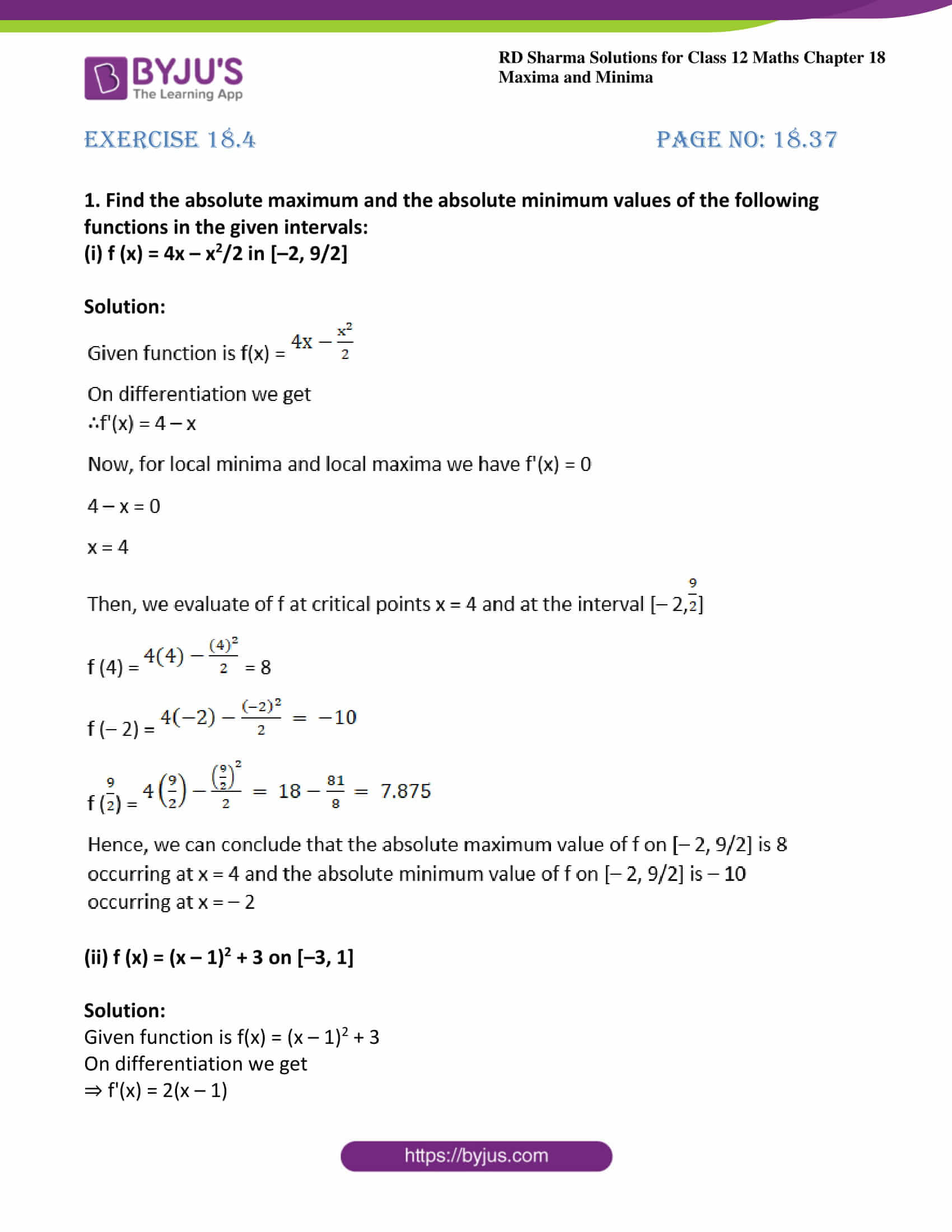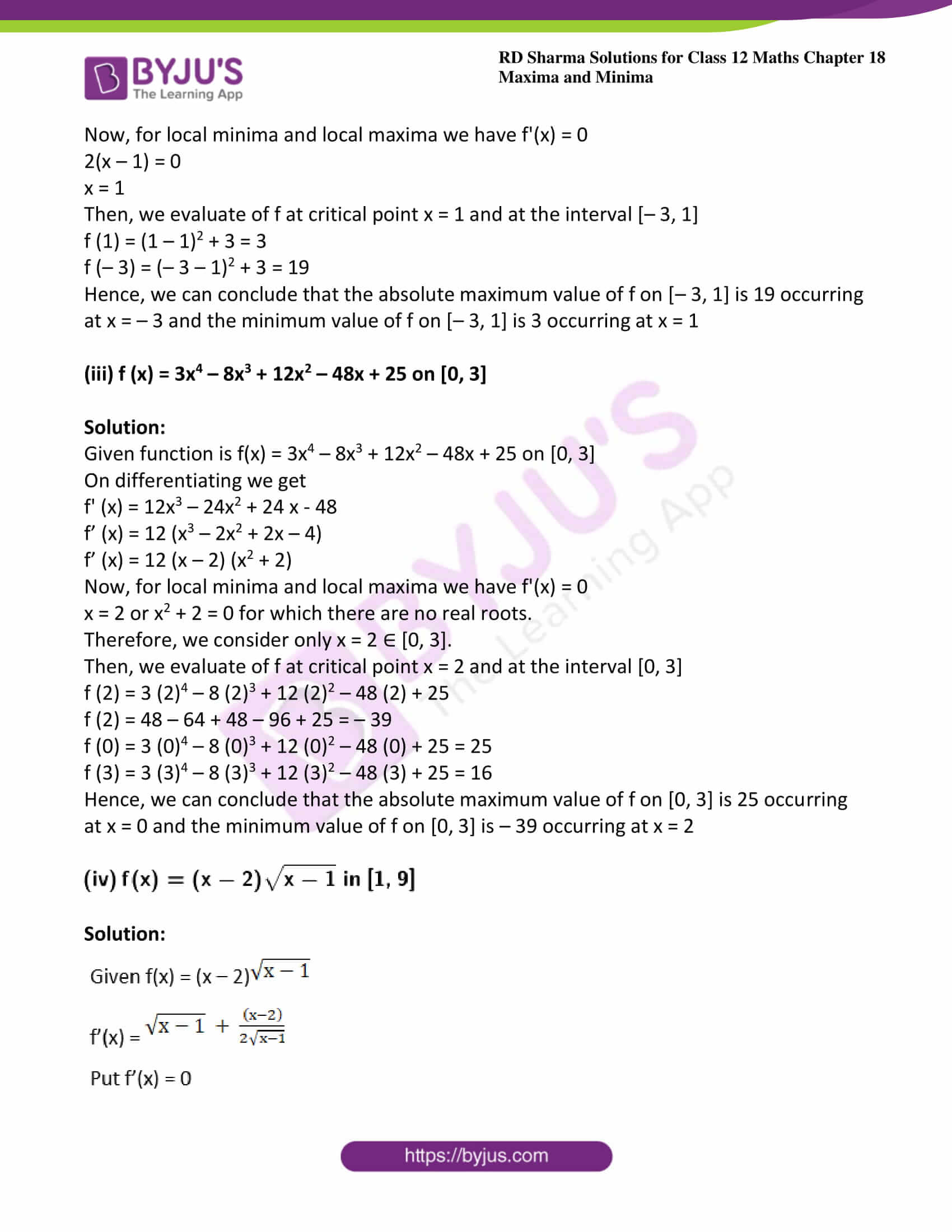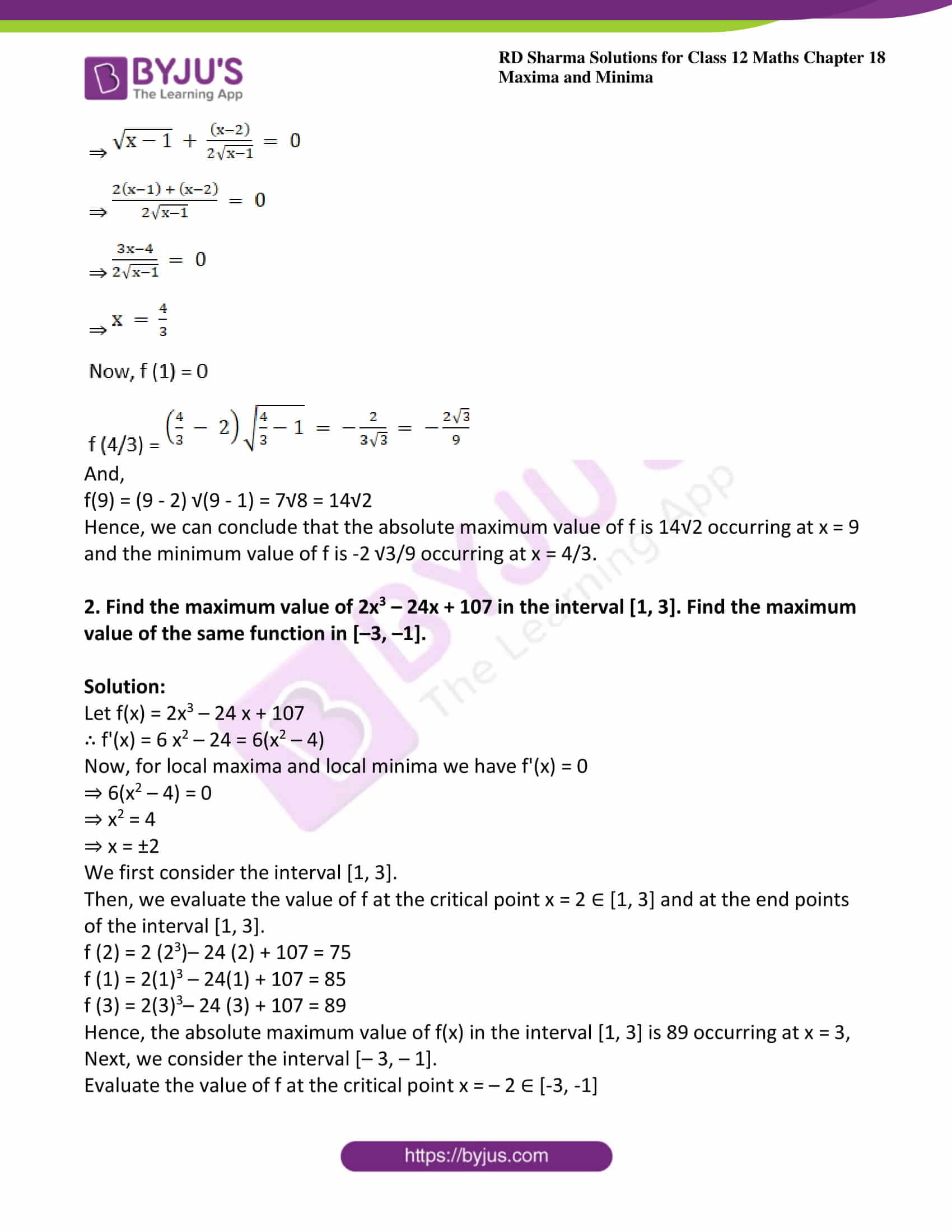### Access other exercises of RD Sharma Solutions For Class 12 Chapter 18 – Maxima and Minima

Exercise 18.1 Solutions

Exercise 18.2 Solutions

Exercise 18.3 Solutions

Exercise 18.5 Solutions

### Exercise 18.4 Page No: 18.37

1. Find the absolute maximum and the absolute minimum values of the following functions in the given intervals:

(i) f (x) = 4x – x2/2 in [–2, 9/2]

Solution: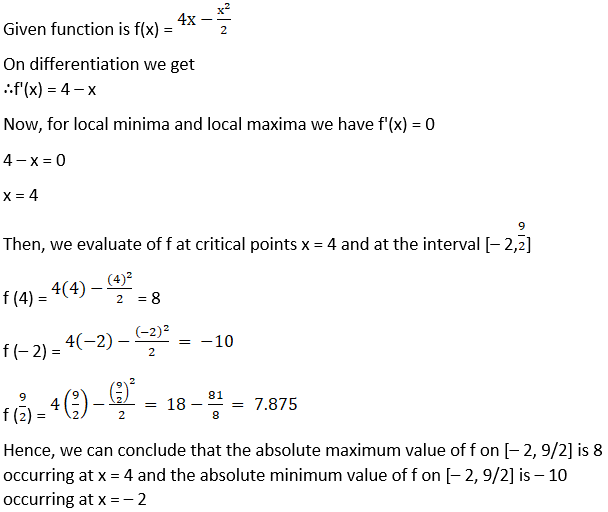(ii) f (x) = (x – 1)2 + 3 on [–3, 1]

Solution:

Given function is f(x) = (x – 1)2 + 3

On differentiation we get

⇒ f'(x) = 2(x – 1)

Now, for local minima and local maxima we have f'(x) = 0

2(x – 1) = 0

x = 1

Then, we evaluate of f at critical point x = 1 and at the interval [– 3, 1]

f (1) = (1 – 1)2 + 3 = 3

f (– 3) = (– 3 – 1)2 + 3 = 19

Hence, we can conclude that the absolute maximum value of f on [– 3, 1] is 19 occurring at x = – 3 and the minimum value of f on [– 3, 1] is 3 occurring at x = 1

(iii) f (x) = 3x4 – 8x3 + 12x2 – 48x + 25 on [0, 3]

Solution:

Given function is f(x) = 3x4 – 8x3 + 12x2 – 48x + 25 on [0, 3]

On differentiating we get

f’ (x) = 12x3 – 24x2 + 24 x – 48

f’ (x) = 12 (x3 – 2x2 + 2x – 4)

f’ (x) = 12 (x – 2) (x2 + 2)

Now, for local minima and local maxima we have f'(x) = 0

x = 2 or x2 + 2 = 0 for which there are no real roots.

Therefore, we consider only x = 2 ∈ [0, 3].

Then, we evaluate of f at critical point x = 2 and at the interval [0, 3]

f (2) = 3 (2)4 – 8 (2)3 + 12 (2)2 – 48 (2) + 25

f (2) = 48 – 64 + 48 – 96 + 25 = – 39

f (0) = 3 (0)4 – 8 (0)3 + 12 (0)2 – 48 (0) + 25 = 25

f (3) = 3 (3)4 – 8 (3)3 + 12 (3)2 – 48 (3) + 25 = 16

Hence, we can conclude that the absolute maximum value of f on [0, 3] is 25 occurring at x = 0 and the minimum value of f on [0, 3] is – 39 occurring at x = 2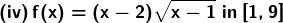Solution: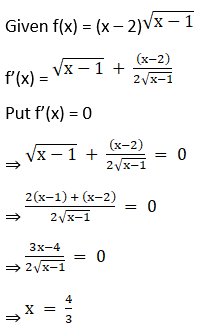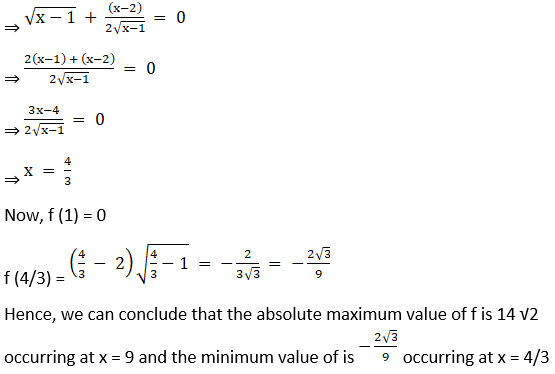And,

f(9) = (9 – 2) √(9 – 1) = 7√8 = 14√2

Hence, we can conclude that the absolute maximum value of f is 14√2 occurring at x = 9 and the minimum value of f is -2 √3/9 occurring at x = 4/3.

2. Find the maximum value of 2x3 – 24x + 107 in the interval [1, 3]. Find the maximum value of the same function in [–3, –1].

Solution:

Let f(x) = 2x3 – 24 x + 107

∴ f'(x) = 6 x2 – 24 = 6(x2 – 4)

Now, for local maxima and local minima we have f'(x) = 0

⇒ 6(x2 – 4) = 0

⇒ x2 = 4

⇒ x = ±2

We first consider the interval [1, 3].

Then, we evaluate the value of f at the critical point x = 2 ∈ [1, 3] and at the end points of the interval [1, 3].

f (2) = 2 (23)– 24 (2) + 107 = 75

f (1) = 2(1)3 – 24(1) + 107 = 85

f (3) = 2(3)3– 24 (3) + 107 = 89

Hence, the absolute maximum value of f(x) in the interval [1, 3] is 89 occurring at x = 3,

Next, we consider the interval [– 3, – 1].

Evaluate the value of f at the critical point x = – 2 ∈ [-3, -1]

f (-3) = 2 (-3)3 – 24 (-3) + 107 = 125

f (-2) = 2 (-2)3 – 24 (-3) + 107 = 139

f (-1) = 2 (-1)3 – 24 (-2) + 107 = 129

Hence, the absolute maximum value of f is 139 and occurs when x = -2.